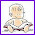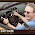### Pi is a ratio, yet irrational?

Here's an interesting dilemma:

We know Pi is an irrational number; mathematicians have proven it to be so.

But its definition says that it is a RATIO of the circumference and the diameter of any circle.

Now, when you divide a rational number by another rational number, you get a rational number.

Doesn't this seem like a contradiction?

In the words of a certain visitor to my site:

In the equation where the circumference is divided by the diameter, when the circumference and diameter are rational values, why is it that the quotient can be an irrational quantity?

The solution

First of all, like a commenter pointed out, Pi being a ratio of two numbers does not mean it is rational. Pi has been established as irrational, and we know
Pi = C/d, where C is the circumference and d is the diameter of some circle.

It follows that either C or d or both have to be irrational!

This is kind of amazing to think about, but it's true: for any circle, either the circumference, or the diameter, or both are irrational (in the abstract world of mathematics...).

BUT (and herein lies the crux of the apparent contradiction)... in the real world where we have to use measurements, of course your measurements will always be rational numbers. ANY measurement you make is just an approximation anyway, and is rational, such as 14.52 cm or 8 1/16 in.

And, if you try to find the value of Pi using measurements from real world, all you get is a rational approximation to the value of Pi.

Even your calculator just gives you a rational approximation for Pi, to however many decimal digits it can show it. But that's all that is necessary for real world applications.mathmom said…
I believe that the solution to this mystery lies in the fact that the circumference and diameter of any given circle cannot both be rational numbers.Unknown said…
Absolutely correct. If C = pi*d, it follows that pi = C/d. Since pi is irrational C and d cannot both be rational. However, it is possible for both C and d to be IRrational.

If d = x where x is rational (e.g., let x = sqr(2)), then C = sqr(2)* pi, the product of which is irrational.

On the other hand, if C is rational (e.g., let C = 2) then d = C/pi = 2/pi which is, of course, irrational.James said…
Essentially the issue here is in how you are defining the term "Irrational Number". Typically the set of irrationals is defined as the set of real numbers not belonging to the set of rational numbers. In turn, rationals are defined as the set of reals that can be written as the ratio of two integers, excluding of course zero in the denominator. Not all ratios are rational!(x, why?) said…
The definition of Irrational Number escaped some students at my school. On a state exam, the question stated "Give an example of an irrational number and explain why it is irrational."

More than one student wrote 22/7, not understanding that that was not pi but only a rough approximation. Nor did they realize that 22/7 is a ratio and therefore a rational number.Anonymous said…
I agree with the practical aspect of "pi". Unless we are dealing with precision, "pi" need not be that accurate. Sufficient decimal point placing can be adjusted to suit the application of this "pi". I believe it is the knowledge in using "pi" that matters, and where actual learning takes place. Many students of mine always ask about how many decimal places they need to use for computation. "Pi" is therefore a good starting point for them.
From: Principle of MathematicsAnonymous said…
"Pi Day" 2008

Hello,

A ratio can be expressed
as a:b, regardless of
[ir]rational nature of
a or b, or both. Hence,
ratios involving pi or
any other irrational
quantities can be mani-
pulated like any other
ratios, e.g., comparing
absolute values, solving
proportions, usage for
similar geometric figures,
etc.

-John Morse